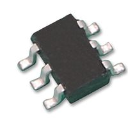# Best way to interface TTL and CMOS levels to processor with VDD=5V?

cancel
Showing results for
Did you mean:
SOLVED

## Best way to interface TTL and CMOS levels to processor with VDD=5V?

3,512 ViewsContributor I

I am busy interfacing things to the inputs of a microcontroller.
If I look at the datasheet for the So8DZ128, in the Appendix A, Electrical Characteristics, DC Characteristics, I find the following:

Input high voltage; all digital inputs,
VIH (input high) for 5V = 0.65 x VDD

VIH (input high) for 3V = 0.7 x VDD

So I interpret this for a 5V supply that the input must be at least 3.25V to register as a logic 1.

Therefore all TTL or 3.3V CMOS devices (Vout high = 2.4V) will need a level-shifter before connecting to the input of the CPU.

I am currently using a Schmitt trigger/buffer to do this (NC7WZ17). The buffer is connected to a supply of 5V.

(And for the reverse direction the same buffer is used, connected to 3.3V)

I know that the inputs of the microcontroller have a pull-up resistor that can be enabled via software, but I do not think this is of much help in this case, only if you connect the controller input to something that is floating (high impedance).

Is this the necessary and correct/best/easiest way to do level shifting ? (and correct me if I am wrong).
What cheap/commonly available integrated circuits do you use to do TTL/CMOS level-shifting for your microcontroller application ?

Thanks

Labels (1)
• ### General

1 Solution
539 ViewsSpecialist III

Hello,

Depending on your switching speed requirement, there may be simpler methods of achieving CMOS to CMOS interface.

For 3.3 volt output to 5 volt input, a series diode can be fitted between the two pins, with output to cathode.  This is in conjunction with enabling the input pullup, or possibly using a lower value external pullup resistor, for greater speed.  The diode would remain conducting during both low and high logic states, and would increase both low and high logic levels by about 0.7 volts.  So 0 -> 3.3 volts at the CMOS output would translate to 0.7 -> 4.0 volts at the input pin, which should provide satisfactory switching for a Vdd of 5 volts.

For 5 volt output to 3.3 volts input, exactly the same series diode method could be used.  In this case, the diode would conduct only during the logic low state.  Here, the diode will prevent a voltage in excess of 3.3 volts reaching the input pin.  Another method would be the use of a simple resistive voltage divider (with input pullup disabled), to reduce the voltage swing.

With the series diode method, the speed limitation is because the input pin is not actively driven to the high state when the diode is non-conducting, and the transition period will depend on the RC time constant caused by the pin input capacitance and the pullup resistor.  Should a substantially faster transition be needed, the provision of an actively driven input would then require the use of a separate buffer device.  A buffer from the 74HCT CMOS family (with 5 volt Vdd}, can provide inputs that are compatible with a 3.3 volt output swing.

However, before including additional interface circuitry, check to see whether the input to a 5 volt peripheral is directly compatible with a 3.3 volt MCU output.  For example, this is true of some LCD displays.

For a "true" TTL output connected to an input of a 5 volt MCU, use an additional 4k7 pullup resistor.  This should raise the TTL output voltage to a sufficient degree.  With a 3.3 volt MCU, the TTL levels should be compatible.

Regards,

Mac

540 ViewsSpecialist III

Hello,

Depending on your switching speed requirement, there may be simpler methods of achieving CMOS to CMOS interface.

For 3.3 volt output to 5 volt input, a series diode can be fitted between the two pins, with output to cathode.  This is in conjunction with enabling the input pullup, or possibly using a lower value external pullup resistor, for greater speed.  The diode would remain conducting during both low and high logic states, and would increase both low and high logic levels by about 0.7 volts.  So 0 -> 3.3 volts at the CMOS output would translate to 0.7 -> 4.0 volts at the input pin, which should provide satisfactory switching for a Vdd of 5 volts.

For 5 volt output to 3.3 volts input, exactly the same series diode method could be used.  In this case, the diode would conduct only during the logic low state.  Here, the diode will prevent a voltage in excess of 3.3 volts reaching the input pin.  Another method would be the use of a simple resistive voltage divider (with input pullup disabled), to reduce the voltage swing.

With the series diode method, the speed limitation is because the input pin is not actively driven to the high state when the diode is non-conducting, and the transition period will depend on the RC time constant caused by the pin input capacitance and the pullup resistor.  Should a substantially faster transition be needed, the provision of an actively driven input would then require the use of a separate buffer device.  A buffer from the 74HCT CMOS family (with 5 volt Vdd}, can provide inputs that are compatible with a 3.3 volt output swing.

However, before including additional interface circuitry, check to see whether the input to a 5 volt peripheral is directly compatible with a 3.3 volt MCU output.  For example, this is true of some LCD displays.

For a "true" TTL output connected to an input of a 5 volt MCU, use an additional 4k7 pullup resistor.  This should raise the TTL output voltage to a sufficient degree.  With a 3.3 volt MCU, the TTL levels should be compatible.

Regards,

Mac